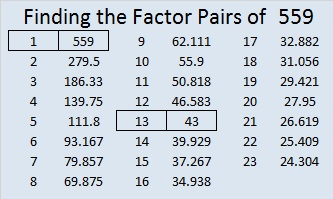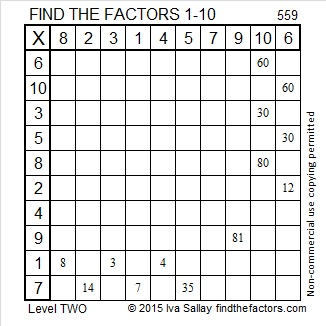# 559 and Level 2

All the prime numbers from 67 to 97 add up to 559, and all the prime numbers from 103 to 127 also add up to 559.

559 is also the hypotenuse of Pythagorean triple 215-516-559. What is the greatest common factor of those three numbers?Print the puzzles or type the solution on this excel file: 10 Factors 2015-07-20

—————————————————————————————————

• 559 is a composite number.
• Prime factorization: 559 = 13 x 43
• The exponents in the prime factorization are 1 and 1. Adding one to each and multiplying we get (1 + 1)(1 + 1) = 2 x 2 = 4. Therefore 559 has exactly 4 factors.
• Factors of 559: 1, 13, 43, 559
• Factor pairs: 559 = 1 x 559 or 13 x 43
• 559 has no square factors that allow its square root to be simplified. √559 ≈ 23.64318—————————————————————————————————1.Paula Beardell Krieg
2.ivasallay